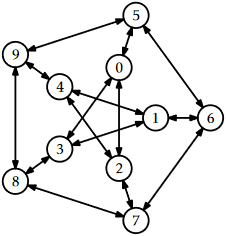# 12.4: Discussion and Exercises

$$\newcommand{\vecs}{\overset { \rightharpoonup} {\mathbf{#1}} }$$ $$\newcommand{\vecd}{\overset{-\!-\!\rightharpoonup}{\vphantom{a}\smash {#1}}}$$$$\newcommand{\id}{\mathrm{id}}$$ $$\newcommand{\Span}{\mathrm{span}}$$ $$\newcommand{\kernel}{\mathrm{null}\,}$$ $$\newcommand{\range}{\mathrm{range}\,}$$ $$\newcommand{\RealPart}{\mathrm{Re}}$$ $$\newcommand{\ImaginaryPart}{\mathrm{Im}}$$ $$\newcommand{\Argument}{\mathrm{Arg}}$$ $$\newcommand{\norm}{\| #1 \|}$$ $$\newcommand{\inner}{\langle #1, #2 \rangle}$$ $$\newcommand{\Span}{\mathrm{span}}$$ $$\newcommand{\id}{\mathrm{id}}$$ $$\newcommand{\Span}{\mathrm{span}}$$ $$\newcommand{\kernel}{\mathrm{null}\,}$$ $$\newcommand{\range}{\mathrm{range}\,}$$ $$\newcommand{\RealPart}{\mathrm{Re}}$$ $$\newcommand{\ImaginaryPart}{\mathrm{Im}}$$ $$\newcommand{\Argument}{\mathrm{Arg}}$$ $$\newcommand{\norm}{\| #1 \|}$$ $$\newcommand{\inner}{\langle #1, #2 \rangle}$$ $$\newcommand{\Span}{\mathrm{span}}$$$$\newcommand{\AA}{\unicode[.8,0]{x212B}}$$

The running times of the depth-first-search and breadth-first-search algorithms are somewhat overstated by the Theorems 12.3.1 and 12.3.2. Define $$\mathtt{n}_{\mathtt{r}}$$ as the number of vertices, $$\mathtt{i}$$, of $$G$$, for which there exists a path from $$\mathtt{r}$$ to $$\mathtt{i}$$. Define $$\mathtt{m}_\mathtt{r}$$ as the number of edges that have these vertices as their sources. Then the following theorem is a more precise statement of the running times of the breadth-first-search and depth-first-search algorithms. (This more refined statement of the running time is useful in some of the applications of these algorithms outlined in the exercises.)

Theorem $$\PageIndex{1}$$

When given as input a Graph, $$\mathtt{g}$$, that is implemented using the AdjacencyLists data structure, the $$\mathtt{bfs(g,r)}$$, $$\mathtt{dfs(g,r)}$$ and $$\mathtt{dfs2(g,r)}$$ algorithms each run in $$O(\mathtt{n}_{\mathtt{r}}+\mathtt{m}_{\mathtt{r}})$$ time.

Breadth-first search seems to have been discovered independently by Moore  and Lee  in the contexts of maze exploration and circuit routing, respectively.

Adjacency-list representations of graphs were presented by Hopcroft and Tarjan  as an alternative to the (then more common) adjacency-matrix representation. This representation, as well as depth-first-search, played a major part in the celebrated Hopcroft-Tarjan planarity testing algorithm that can determine, in $$O(\mathtt{n})$$ time, if a graph can be drawn, in the plane, and in such a way that no pair of edges cross each other .

In the following exercises, an undirected graph is one in which, for every $$\mathtt{i}$$ and $$\mathtt{j}$$, the edge $$(\mathtt{i},\mathtt{j})$$ is present if and only if the edge $$(\mathtt{j},\mathtt{i})$$ is present.

Exercise $$\PageIndex{1}$$

Draw an adjacency list representation and an adjacency matrix representation of the graph in Figure $$\PageIndex{1}$$.Figure $$\PageIndex{1}$$: An example graph.

Exercise $$\PageIndex{2}$$

The incidence matrix representation of a graph, $$G$$, is an $$\mathtt{n}\times\mathtt{m}$$ matrix, $$A$$, where

$\displaystyle A_{i,j} = \begin{cases} -1 & \text{if vertex i the source of edge j} \\ +1 & \text{if vertex i the target of edge j} \\ 0 & \text{otherwise.} \end{cases}\nonumber$

1. Draw the incident matrix representation of the graph in Figure $$\PageIndex{1}$$.
2. Design, analyze and implement an incidence matrix representation of a graph. Be sure to analyze the space, the cost of $$\mathtt{addEdge(i,j)}$$, $$\mathtt{removeEdge(i,j)}$$, $$\mathtt{hasEdge(i,j)}$$, $$\mathtt{inEdges(i)}$$, and $$\mathtt{outEdges(i)}$$.

Exercise $$\PageIndex{3}$$

Illustrate an execution of the $$\mathtt{bfs(G,0)}$$ and $$\mathtt{dfs(G,0)}$$ on the graph, $$\mathtt{G}$$, in Figure $$\PageIndex{1}$$.

Exercise $$\PageIndex{4}$$

Let $$G$$ be an undirected graph. We say $$G$$ is connected if, for every pair of vertices $$\mathtt{i}$$ and $$\mathtt{j}$$ in $$G$$, there is a path from $$\mathtt{i}$$ to $$\mathtt{j}$$ (since $$G$$ is undirected, there is also a path from $$\mathtt{j}$$ to $$\mathtt{i}$$). Show how to test if $$G$$ is connected in $$O(\mathtt{n}+\mathtt{m})$$ time.

Exercise $$\PageIndex{5}$$

Let $$G$$ be an undirected graph. A connected-component labelling of $$G$$ partitions the vertices of $$G$$ into maximal sets, each of which forms a connected subgraph. Show how to compute a connected component labelling of $$G$$ in $$O(\mathtt{n}+\mathtt{m})$$ time.

Exercise $$\PageIndex{6}$$

Let $$G$$ be an undirected graph. A spanning forest of $$G$$ is a collection of trees, one per component, whose edges are edges of $$G$$ and whose vertices contain all vertices of $$G$$. Show how to compute a spanning forest of of $$G$$ in $$O(\mathtt{n}+\mathtt{m})$$ time.

Exercise $$\PageIndex{7}$$

We say that a graph $$G$$ is strongly-connected if, for every pair of vertices $$\mathtt{i}$$ and $$\mathtt{j}$$ in $$G$$, there is a path from $$\mathtt{i}$$ to $$\mathtt{j}$$. Show how to test if $$G$$ is strongly-connected in $$O(\mathtt{n}+\mathtt{m})$$ time.

Exercise $$\PageIndex{8}$$

Given a graph $$G=(V,E)$$ and some special vertex $$\mathtt{r}\in V$$, show how to compute the length of the shortest path from $$\mathtt{r}$$ to $$\mathtt{i}$$ for every vertex $$\mathtt{i}\in V$$.

Exercise $$\PageIndex{9}$$

Give a (simple) example where the $$\mathtt{dfs(g,r)}$$ code visits the nodes of a graph in an order that is different from that of the $$\mathtt{dfs2(g,r)}$$ code. Write a version of $$\mathtt{dfs2(g,r)}$$ that always visits nodes in exactly the same order as $$\mathtt{dfs(g,r)}$$. (Hint: Just start tracing the execution of each algorithm on some graph where $$\mathtt{r}$$ is the source of more than 1 edge.)

Exercise $$\PageIndex{10}$$

A universal sink in a graph $$G$$ is a vertex that is the target of $$\mathtt{n}-1$$ edges and the source of no edges.1 Design and implement an algorithm that tests if a graph $$G$$, represented as an AdjacencyMatrix, has a universal sink. Your algorithm should run in $$O(\mathtt{n})$$ time.

## Footnotes

1A universal sink, $$\mathtt{v}$$, is also sometimes called a celebrity: Everyone in the room recognizes $$\mathtt{v}$$, but $$\mathtt{v}$$ doesn't recognize anyone else in the room.

This page titled 12.4: Discussion and Exercises is shared under a CC BY license and was authored, remixed, and/or curated by Pat Morin (Athabasca University Press) .